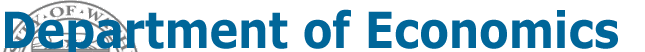Home
Syllabus
Homework
Notes
Announcements
Project
Software

Eric Zivot Economics 581 Econometric Theory II

Winter 2001

Last updated: February 12, 2001

Notes: H denotes "Hyashi" and B denotes "Berndt". The reading assignments are grouped by topic. The topics roughly correspond with the weeks of the quarter but some topics may more or less than a week to complete.

Least Squares

• H, chapter 1 (sections 1-4, 6-7)
• Berndt, The Practice of Econometrics, chapter 2 (Capital Asset Pricing Model) and 3 (estimation of cost functions and learning curves).

Introduction to Asymptotics

• H, chapter 2 (section 1)

Midterm Exam

Time Series Concepts

• H, chapter 2 (section 2)

Asymptotics for Regression

• H, chapter 2  (sections 3-8, 10-11)

Regression with Engodenous Variables